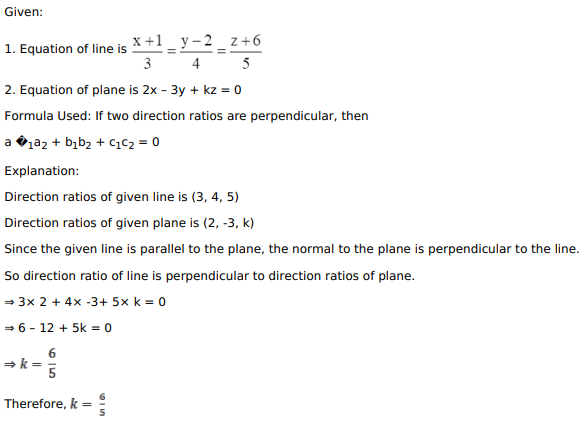# Mark against the correct answer in each of the following:

Question:

Mark against the correct answer in each of the following:

If the line $\frac{x+1}{3}=\frac{y-2}{4}=\frac{z+6}{5}$ is parallel to the plane $2 x-3 y+k z=0$, then the value of $k$ is

A. $\frac{5}{6}$

B. $\frac{6}{5}$

C. $\frac{3}{4}$

D. $\frac{4}{5}$

Solution: